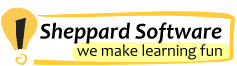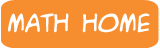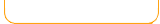Place Value
 TutorialPlace Value ModelsInteractive tutorial. Models help you learn place value with this fruit shoot game. Expanded Form Underlined Digit Value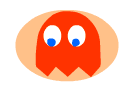Math Man Chase the correct answers through a maze. E.G. 200 + 10+3 = 213. What is the value of the underlined digit? In the example, the value is 20. Identify The Digit Compare Numbers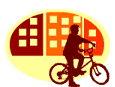Scooter Quest 1 Identify where a given digit is. For example, which of these three numbers has a 3 in the ones digit? 104, 125, or 163? Answer - 163. Use place value to help you compare numbers up to ten million with this Fruit Shoot game. Use place value to help you order numbers up to one million with this Balloon pop game. Chase ghosts through a maze to answer value of a digit questions. Different Ways Of Showing A Number Identify different ways of showing a number. For example, 103 is either 1 hundred + 3 ones or 10 tens +3 ones.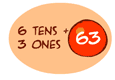Place Value - Expanded Form Shoot the fruit that has the correct number for the expanded form. For example, 6 tens and 3 ones = 63 Shoot the fruit that has the correct number for the expanded form. For example, 8 ones and 2 tenths = 8.2